Urban Street Network Analysis in a Computational Notebook

REGION Special Issue: The Potential of Notebooks for Scientific Publication, Reproducibility and Dissemination

Geoff Boeing

University of Southern California

Los Angeles, USA

# Abstract¶

Computational notebooks offer researchers, practitioners, students, and educators the ability to interactively conduct analytics and disseminate reproducible workflows that weave together code, visuals, and narratives. This article explores the potential of computational notebooks in urban analytics and planning, demonstrating their utility through a case study of OSMnx and its tutorials repository. OSMnx is a Python package for working with OpenStreetMap data and modeling, analyzing, and visualizing street networks anywhere in the world. Its official demos and tutorials are distributed as open-source Jupyter notebooks on GitHub. This article showcases this resource by documenting the repository and demonstrating OSMnx interactively through a synoptic tutorial adapted from the repository. It illustrates how to download urban data and model street networks for various study sites, compute network indicators, visualize street centrality, calculate routes, and work with other spatial data such as building footprints and points of interest. Computational notebooks help introduce methods to new users and help researchers reach broader audiences interested in learning from, adapting, and remixing their work. Due to their utility and versatility, the ongoing adoption of computational notebooks in urban planning, analytics, and related geocomputation disciplines should continue into the future.

Keywords: Computational Notebook, Jupyter, OpenStreetMap, OSMnx, Python, Street Network, Urban Planning

# Introduction¶

A traditional academic and professional divide has long existed between code creators and code users. The former would develop software tools and workflows for professional or research applications, which the latter would then use to conduct analyses or answer scientific questions. Today, however, these boundary lines increasingly blur as computation percolates throughout both the natural and social sciences. As quantitatively-oriented academics gradually shift away from monolithic, closed-source data analysis software systems like SPSS and ArcGIS, they increasingly embrace coding languages like R and Python to script and document their research workflows (Padgham et al. 2019). Developing shareable, reproducible, and recomputable scripts in R or Python to acquire, transform, describe, visualize, and model data, these researchers act as both code creators and code users.

An important trend in this methodological trajectory has been the widespread adoption of the computational notebook. A computational notebook is a computer file that replaces the traditional lab notebook and intersperses plain-language narrative, hyperlinks, and images with snippets of code in the paradigm of literate programming (Knuth 1992). These notebooks are easily distributed and integrate well with version control systems like Git because they are simply structured text files. They have pedagogical value in introducing students to computational thinking and coding techniques while thoroughly explaining each new programming language facet as it is introduced. They also offer research value in documenting data, questions, hypotheses, procedures, experiments, and results in detail alongside each's attendant computations (Pérez and Granger 2007; Kluyver et al. 2016).

Computational notebooks thus open up the world of analytics to a wider audience than was possible in the past. This particularly impacts disciplines that encompass diverse methodologies and skillsets. For example, urban planning, like many academic domains related or adjacent to regional science, comprises a broad set of scholars, students, and working professionals with a wide range of computational aptitude. Some urban planners focus on policymaking within the political constraints of city hall. Others employ qualitative methods to work in and with vulnerable communities. Others develop simulation models to forecast urbanization patterns and infrastructure needs. Others intermingle these, and many more, different approaches to understanding and shaping the city. Yet all urban planners benefit from basic quantitative literacy and an ability to reason critically with data. This scholarly and professional imperative aligns with the growing importance of computational thinking in the urban context and parallel trends in geocomputation (Harris et al. 2017), geographic data science (Kang et al. 2019; Poorthuis and Zook 2019; Singleton and Arribas-Bel 2019), and the open-source/open-science movements (Rey 2019).

Urban planning and its related disciplines benefit accordingly from the growing adoption of computational notebooks in pedagogy, research, and practice. Computation is increasingly central to the field and its practitioners benefit from open and reproducible approaches to analyzing urban data and predicting city futures (Kedron et al. 2019; Kontokosta 2018; Batty 2019). In the Python universe, for example, numerous new tools now exist to support urban analytics and planning processes, including data wrangling/analysis (pandas), visualization (matplotlib), geospatial wrangling/analysis (geopandas), spatial data science and econometrics (pySAL), mapping (cartopy), web mapping (folium), network analysis (NetworkX), land use modeling/simulation (UrbanSim), activity-based travel modeling (ActivitySim), and computational notebooks themselves (Jupyter).

Another Python tool useful for urban planning research and practice – and the primary focus of this article – is OSMnx, a package for street network analysis (Boeing 2017). OSMnx allows users to download spatial data (including street networks, other networked infrastructure, building footprints, and points of interest) from OpenStreetMap then model, analyze, and visualize them. To introduce new users to its functionality and capabilities, OSMnx's official demos and tutorials are developed and maintained in Jupyter notebook format. This repository in turn offers a compelling case study of the potential of computational notebooks to document and disseminate geospatial software tools.

This article introduces OSMnx as a computational tool for urban street network analysis by way of these computational notebooks. It describes their repository and highlights examples from them, inline here, to illustrate the use and value of computational notebooks. To do so, it demonstrates how to interactively execute the code in this article itself by using Docker to run a containerized computational environment including Jupyter Lab as an interactive web-based interface. The article is organized as follows. First, it presents the repository containing OSMnx's demo and tutorial notebooks. Then it describes how to run OSMnx's computational environment via Docker. Next it demonstrates the use of OSMnx interactively in the article itself through a synoptic tutorial adapted from this repository. Finally, it concludes by discussing the prospects of notebooks for facilitating the adoption of computational workflows in urban analytics and planning.

# The OSMnx Examples Repository¶

OSMnx's official demos, tutorials, and examples are in Jupyter notebook format in a GitHub repository. The repository's root contains a license file, a readme file, an environment definition file, repository contributing guidelines, and a notebooks folder. Within that folder, the repository contains 19 thematically organized Jupyter notebook files that collectively provide a short self-directed tutorial-style course in using OSMnx. The following notebooks are included there:

1. An introductory survey of features
2. A more comprehensive overview of OSMnx's basic functionality
3. Using OSMnx to produce shapefiles
4. Modeling and visualizing street networks in different places at different scales
5. Using OSMnx's network topology cleaning and simplification features
7. Conducting street network analyses with OSMnx and its NetworkX dependency
8. Visualizing street networks and study sites
9. Working with dual graphs of street networks
10. Producing figure-ground diagrams for urban form analysis
11. Working with building footprints
12. Interactive web mapping of street networks and routes
13. Attaching elevations to the network and calculating street grades
14. Working with isolines and isochrones
15. Cleaning complex street intersections
16. Calculating street bearings
17. Working with other types of spatial infrastructure
18. Visualizing street network orientation with polar histograms
19. Interfacing between OSMnx and igraph for fast algorithm implementations in the C language

This resource is useful for introducing users to the OSMnx software package, demonstrating how to download, model, analyze, and visualize street networks in Python, and illustrating several basic and intermediate spatial network analyses. To run the code examples in this resource repository, one must have access to a Python installation with the code dependencies installed, including Jupyter itself for running the notebook files. Two primary options exist for installing this computational environment. The first is installing Python locally, then configuring it and installing all the necessary packages and dependencies. This can be time-consuming and requires some prior experience beyond the scope of this article. The second, and easier, option is to simply run everything in a pre-built Docker container. This latter option is detailed in the following section.

# The Computational Environment¶

The OSMnx project's reference Docker image contains a stable, consistent computational environment for running OSMnx on any computer. Docker is a virtualization tool that allows complex software stacks to be delivered as self-contained packages called images, allowing users to run software without having to compile or install a complex chain of dependencies. Instead, users install Docker on their computer then tell it to run a certain image as an instance called a container.

This article can be read in its static form (i.e., HTML or PDF) or it can be executed interactively (i.e., via its .ipynb Jupyter notebook file). For interactive execution, install Docker and run the official OSMnx container as follows. First, download and install Docker Desktop. Once it is installed and running on your computer, open Docker's settings/preferences and ensure that your local drives are shared with Docker so the container has access to the notebook file. Then run the OSMnx Docker container (which contains a Python installation and all the packages needed to run OSMnx, including Jupyter Lab) by following the platform-specific instructions below.

If you are on Windows open a command prompt, change directory to the location of this notebook file then run:

docker run --rm -it -p 8888:8888 -v "%cd%":/home/jovyan/work gboeing/osmnx:v10

If you are on Mac/Linux open a terminal window, change directory to the location of this notebook file then run:

docker run --rm -it -p 8888:8888 -v "\$PWD":/home/jovyan/work gboeing/osmnx:v10

Once the container is running per these instructions, open your computer's web browser and visit http://localhost:8888 to access Jupyter Lab and open this article's notebook file.

# Street Network Analysis with OSMnx¶

Here we showcase the resource repository inline to demonstrate potential applications. In particular, we highlight specific material from its notebooks (enumerated above), adapting their code into this interactive article to introduce OSMnx and illustrate some of the capabilities of a computational notebook.

First we import the necessary Python modules:

In :
import matplotlib.cm as cm
import matplotlib.colors as colors
import networkx as nx
from IPython.display import Image
from pprint import pprint


matplotlib is a package for data visualization and plotting. NetworkX is a package for generic network analysis. IPython provides interactive computing and underpins our Python-language Jupyter environment (Pérez and Granger 2007). pprint allows us to "pretty print" Python data structures to make them easier to read inline.

Next we import OSMnx itself, configure it, and display its version number:

In :
import osmnx as ox
ox.config(log_console=True, use_cache=True)
ox.__version__

Out:
'0.10'

The configuration step tells OSMnx to log its actions to the terminal window and to use a cache. This cache saves a local copy of any data downloaded by OSMnx to prevent re-downloading the same data each time the code is run.

Next we use OSMnx to download the street network of Piedmont, California, construct a graph model of it (via NetworkX), then plot the network with the plot_graph function (which uses matplotlib under the hood):

In :
# create a graph of Piedmont's drivable street network then plot it
G = ox.graph_from_place('Piedmont, California, USA', network_type='drive')
fig, ax = ox.plot_graph(G)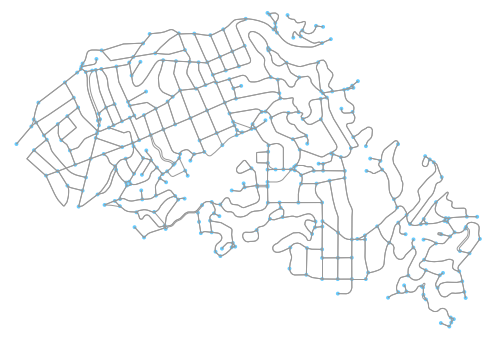In the figure above, the network's intersections and dead-ends (i.e., graph nodes) appear as light blue circles and its street segments (i.e., graph edges) appear as gray lines. This is the street network within the municipal boundaries of the city of Piedmont, California. We select this study site for pedagogical purposes as it is a relatively small, self-contained municipality and lends itself to convenient visualization and indicator calculation here. Note that we specified network_type='drive' so this is specifically the drivable network in the city. OSMnx can also automatically download and model walkable and bikeable street networks by changing this argument.

## Calculating Network Indicators¶

Now that we have a model of the network, we can calculate some statistics and indicators. First, what area does our network cover in square meters? To calculate this, we project the graph, convert its projected nodes to a geopandas GeoDataFrame, then calculate the area of the convex hull of this set of node points in the Euclidean plane:

In :
# project graph then calculate its nodes' convex hull area
G_proj = ox.project_graph(G)
nodes_proj = ox.graph_to_gdfs(G_proj, edges=False)
graph_area_m = nodes_proj.unary_union.convex_hull.area
graph_area_m

Out:
4224782.349449131

Thus, this network covers approximately 4.2 square kilometers. When projecting graphs, OSMnx by default uses the Universal Transverse Mercator (UTM) coordinate system and automatically determines the UTM zone for projection based on the network's centroid. Other coordinate reference systems can be defined by the user to customize this projection behavior.

Next, we compute and inspect some basic stats about the network:

In :
# calculate and print basic network stats
stats = ox.basic_stats(G_proj, area=graph_area_m, clean_intersects=True, circuity_dist='euclidean')
pprint(stats)

{'circuity_avg': 1.11354525174028,
'clean_intersection_count': 271,
'clean_intersection_density_km': 64.1453162753664,
'edge_density_km': 26951.828421373437,
'edge_length_avg': 121.39190724946685,
'edge_length_total': 113865.60899999991,
'intersection_count': 312,
'intersection_density_km': 73.84995822108604,
'k_avg': 5.421965317919075,
'm': 938,
'n': 346,
'node_density_km': 81.89771007851208,
'self_loop_proportion': 0.006396588486140725,
'street_density_km': 14061.652905680734,
'street_length_avg': 121.23963877551029,
'street_length_total': 59407.42300000004,
'street_segments_count': 490,
'streets_per_node_avg': 2.953757225433526,
'streets_per_node_counts': {0: 0, 1: 34, 2: 0, 3: 263, 4: 47, 5: 1, 6: 1},
'streets_per_node_proportion': {0: 0.0,
1: 0.09826589595375723,
2: 0.0,
3: 0.7601156069364162,
4: 0.13583815028901733,
5: 0.002890173410404624,
6: 0.002890173410404624}}


For example, we can see that this network has 346 nodes (n) and 938 edges (m). The streets in this network are 11% more circuitous (circuity_avg) than straight-line would be. The average street segment length is 121 meters (street_length_avg). We can inspect more stats, primarily topological in nature, with the extended_stats function. As the results of many of these indicators are verbose (i.e., calculated at the node-level), we print only the indicators' names here:

In :
# calculate and print extended network stats
more_stats = ox.extended_stats(G, ecc=True, bc=True, cc=True)
for key in sorted(more_stats.keys()):
print(key)

avg_neighbor_degree
avg_neighbor_degree_avg
avg_weighted_neighbor_degree
avg_weighted_neighbor_degree_avg
betweenness_centrality
betweenness_centrality_avg
center
closeness_centrality
closeness_centrality_avg
clustering_coefficient
clustering_coefficient_avg
clustering_coefficient_weighted
clustering_coefficient_weighted_avg
degree_centrality
degree_centrality_avg
diameter
eccentricity
pagerank
pagerank_max
pagerank_max_node
pagerank_min
pagerank_min_node
periphery


The average neighborhood degree indicators refer to the mean degree of nodes in the neighborhood of each node. The centrality indicators (betweenness, closeness, degree, and PageRank) identify how "central" or important each node is to the network in terms of its topological structure. The clustering coefficient indicators represent the extent to which a node's neighborhood forms a complete graph. The extended stats also include the network's eccentricity (the maximum distance from each node to all other nodes), diameter (maximum eccentricity in the network), radius (minimum eccentricity in the network), center (set of all nodes whose eccentricity equals the radius), and periphery (set of all nodes whose eccentricity equals the diameter). Additional information about the various indicators is available online in OSMnx's documentation.

Now that we have modeled the street network and computed various indictors of its geometry and topology, we can finally save our graph to disk as an ESRI shapefile or a GraphML file (an open-source format for graph serialization), allowing easy re-use in other GIS or network analysis software:

In :
# save the network model to disk as a shapefile and graphml
ox.save_graph_shapefile(G, filename='mynetwork_shapefile')
ox.save_graphml(G, filename='mynetwork.graphml')


## Visualizing Street Centrality¶

OSMnx is built on top of NetworkX, a powerful network analysis package developed at Los Alamos National Laboratory (Hagberg et al. 2008). We can use it to calculate and visualize the closeness centrality of different streets in the network. Closeness centrality measures how central a node or edge is in a network and is defined as the reciprocal of the sum of the distance-weighted shortest paths between the node/edge and every other node/edge in the network.

First, we convert our graph to its line graph (sometimes called the dual graph; see Porta et al. ) which inverts its topological definitions such that streets become nodes and intersections become edges. Then we calculate the closeness centrality of each node (i.e., street in the line graph):

In :
# calculate node closeness centrality of the line graph
edge_centrality = nx.closeness_centrality(nx.line_graph(G))


Now that we have calculated the centrality of each street in the network, we visualize it with matplotlib via OSMnx's plot_graph function, using the inferno color map to represent the most-central streets in bright yellow and the least-central streets in dark purple:

In :
# make a list of graph edge centrality values
ev = [edge_centrality[edge + (0,)] for edge in G.edges()]

# create a color scale converted to list of colors for graph edges
norm = colors.Normalize(vmin=min(ev)*0.8, vmax=max(ev))
cmap = cm.ScalarMappable(norm=norm, cmap=cm.inferno)
ec = [cmap.to_rgba(cl) for cl in ev]

# color the edges in the original graph by closeness centrality in line graph
fig, ax = ox.plot_graph(G, bgcolor='black', axis_off=True, node_size=0,
edge_color=ec, edge_linewidth=2, edge_alpha=1)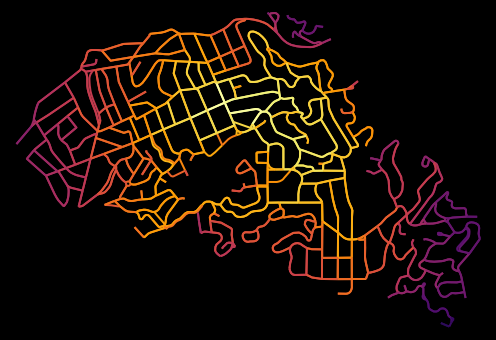## Network Routing¶

OSMnx allows researchers and practitioners to calculate routes and simulate trips along the network using various shortest-path algorithms, such as Dijkstra's (1959). We demonstrate this here. First we use OSMnx to find the network nodes nearest to two latitude-longitude points:

In :
# find the network nodes nearest to two points
orig_node = ox.get_nearest_node(G, (37.825956, -122.242278))
dest_node = ox.get_nearest_node(G, (37.817180, -122.218078))


Next we compute the shortest path between these origin and destination nodes using Dijkstra's algorithm weighted by length (i.e., geometric distance along the street network). Then we use OSMnx to plot this route along the network:

In :
# calculate the shortest path between these nodes then plot it
route = nx.shortest_path(G, orig_node, dest_node, weight='length', method='dijkstra')
fig, ax = ox.plot_graph_route(G, route, node_size=0)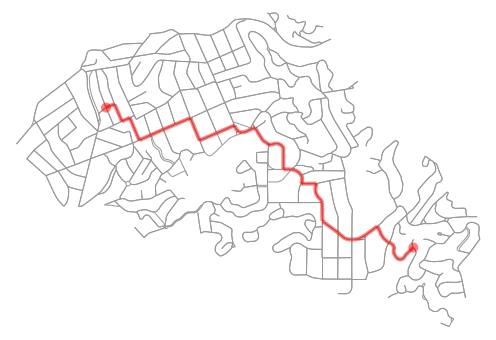Finally, we can calculate some statistics of our route, including its total length, in meters:

In :
# what is the network distance of this route?
net_dist = nx.shortest_path_length(G, orig_node, dest_node, weight='length', method='dijkstra')
net_dist

Out:
3284.0989999999997

Thus, this trip would travel approximately 3.3 kilometers along the network. We can also calculate the straight-line distance between these two network nodes as-the-crow-flies, using OSMnx's vectorized great-circle calculator:

In :
# what is the straight-line distance from origin to destination?
sl_dist = ox.great_circle_vec(G.node[orig_node]['y'], G.node[orig_node]['x'],
G.node[dest_node]['y'], G.node[dest_node]['x'])
sl_dist

Out:
2340.8766018171827

Comparing these two distance values, we can compute an indicator of trip circuity: that is, how much greater the network-constrained distance is between two nodes compared to the straight-line distance between them. In this case, we can see that the network distance is approximately 40% longer than the straight-line distance:

In :
# how much longer is the network distance than the straight-line?
net_dist / sl_dist

Out:
1.4029355487814306

So far, we have modeled and analyzed the street network of Piedmont, California. However, we are not constrained to study sites in the United States. OpenStreetMap is a global mapping project and OSMnx can model networks anywhere in the world, such as Modena, Italy:

In :
# create a graph of Modena's drivable street network then plot it
G = ox.graph_from_place('Modena, Italy', retain_all=True)
fig, ax = ox.plot_graph(G, fig_height=8, node_size=0, edge_linewidth=0.5)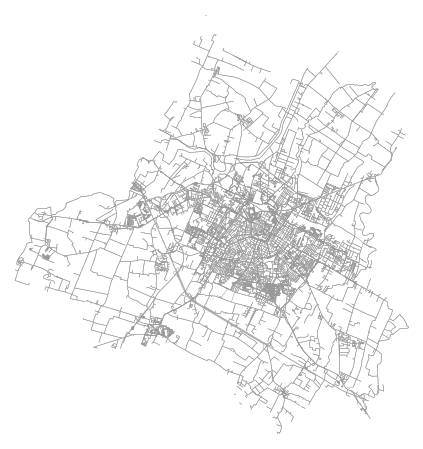We have seen how to download street network data and turn it into a graph-based model using OSMnx's graph_from_place function. This function geocodes the place name using OpenStreetMap's Nominatim web service, identifies its bounding polygon, then downloads all the network data within this polygon from OpenStreetMap's Overpass API. This workflow easily handles well-defined place names. However, OSMnx offers additional functionality to download and model networks for other study sites as well.

For example, if OpenStreetMap does not have a bounding polygon for a specific study site, we can acquire its street network anyway by passing a polygon directly into the graph_from_polygon function. Or we can pass in latitude-longitude coordinates and a distance into the graph_from_point function as demonstrated here, where we visualize the network within a bounding box around the University of California, Berkeley's Wurster Hall:

In :
# create a graph around UC Berkeley then plot it
wurster_hall = (37.870605, -122.254830)
one_mile = 1609 #one mile in meters
G = ox.graph_from_point(wurster_hall, distance=one_mile, network_type='drive')
fig, ax = ox.plot_graph(G, node_size=0)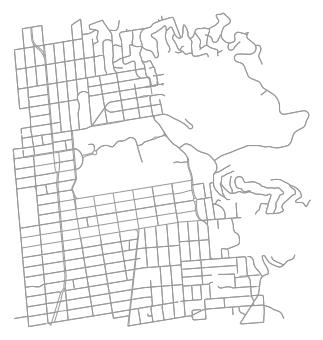OSMnx also accepts place queries as unambiguous Python dictionaries to help the geocoder find a specific matching study site when several names might approximately overlap. In this example, we download the street network of San Francisco, California by defining the query with such a dictionary:

In :
# create a graph of San Francisco's drivable street network then plot it
place = {'city'   : 'San Francisco',
'state'  : 'California',
'country': 'USA'}
G = ox.graph_from_place(place, network_type='drive')


All of the preceding examples have focused on urban and suburban street networks. However, OSMnx can also download and model other networked infrastructure types by passing in custom queries via the infrastructure argument. Such networked infrastructure could include power lines, the canal systems of Venice or Amsterdam, or the New York City subway's rail infrastructure as illustrated in this example:

In :
# create a graph of NYC's subway rail infrastructure then plot it
G = ox.graph_from_place('New York City, New York, USA',
retain_all=False, truncate_by_edge=True, simplify=True,
network_type='none', infrastructure='way["railway"~"subway"]')

fig, ax = ox.plot_graph(G, node_size=0)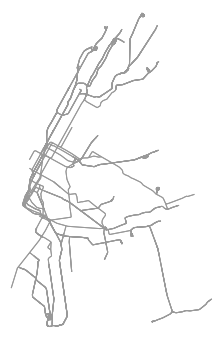Note that the preceding code snippet modeled subway rail infrastructure which thus includes crossovers, sidings, spurs, yards, and the like. For a station-based train network model, the analyst would be best-served downloading and modeling a station adjacency matrix.

Beyond networked infrastructure, OSMnx can also work with OpenStreetMap building footprint and points of interest data. For example, we can download and visualize the building footprints near New York's Empire State Building:

In :
# download and visualize the building footprints around the empire state bldg
point = (40.748482, -73.985402) #empire state bldg coordinates
dist = 812 #meters
gdf = ox.footprints_from_point(point=point, distance=dist)
gdf_proj = ox.project_gdf(gdf)
bbox_proj = ox.bbox_from_point(point=point, distance=dist, project_utm=True)
fig, ax = ox.plot_footprints(gdf_proj, bbox=bbox_proj, bgcolor='#333333', color='w', figsize=(6,6))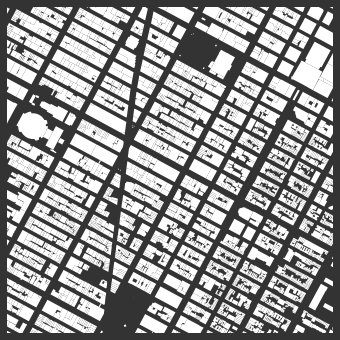Finally, we can download and inspect the amenities matching the tag "restaurants" near the Empire State Building and then display the five most common cuisine types

In :
# download restaurants near the empire state bldg then display them
gdf = ox.pois_from_point(point=point, distance=dist, amenities=['restaurant'])

Out:
name cuisine
357620442 Dolcino Trattoria Toscana italian
419359995 Little Alley chinese
419367625 Ramen Takumi japanese;ramen
561042187 Les Halles french
663104998 Tick Tock Diner diner
In :
# show the five most common cuisine types among these restaurants

Out:
indian      22
korean      15
italian     14
japanese    13
pizza        9
Name: cuisine, dtype: int64

# Conclusion¶

This article argued that computational notebooks underpin an important emerging pillar in urban analytics and planning research, pedagogy, and practice. To demonstrate this, it presented the official repository of computational notebooks that the OSMnx project uses for tutorials, demos, and guides. It illustrated the use of these notebooks by highlighting specific examples from them, inline and interactively within this article, as an introduction to this modeling and analysis software. OSMnx itself is a Python package for downloading, modeling, analyzing, and visualizing data from OpenStreetMap. It lets users analyze networked infrastructure like street networks as well as building footprints, points of interest, elevation data, and more. This article demonstrated how computational notebooks can provide a tutorial-style introduction to scientific software such as this.

The OSMnx project uses computational notebooks because they offer several advantages. First, they empower scientific reproducibility, replication, sharing, and remixing. Second, they allow researchers to intermingle data analyses with visualizations and narratives to ask and answer research questions. Third, they offer "follow-along" guides for introducing software and methods to new users, such as in this repository for OSMnx or even in the university classroom. Finally, they help researchers reach a wider community of interest by making their methodologies and analyses more legible to a broad audience potentially interested in adapting and remixing their work. For these reasons and more, we expect to see growing adoption of computational notebooks in the urban planning discipline and related analytics fields.

# Appendix¶

The interested reader may consult the following web sites for more information and resources as discussed in the article:

Batty, M. (2019). Urban Analytics Defined. Environment and Planning B: Urban Analytics and City Science, 46(3), 403–405. https://doi.org/10.1177/2399808319839494

Boeing, G. (2017). OSMnx: New Methods for Acquiring, Constructing, Analyzing, and Visualizing Complex Street Networks. Computers, Environment and Urban Systems, 65, 126–139. https://doi.org/10.1016/j.compenvurbsys.2017.05.004

Dijkstra, E. W. (1959). A Note on Two Problems in Connexion with Graphs. Numerische Mathematik, 1(1), 269–271. https://doi.org/10.1007/BF01386390

Hagberg, A. A., Schult, D. A., & Swart, P. J. (2008). Exploring Network Structure, Dynamics, and Function using NetworkX. In G. Varoquaux, T. Vaught, & J. Millman (Eds.), Proceedings of the 7th Python in Science Conference (pp. 11–16). Pasadena, California: SciPy.

Harris, R., O'Sullivan, D., Gahegan, M., Charlton, M., Comber, L., Longley, P., … Evans, A. (2017). More Bark than Bytes? Reflections on 21+ Years of Geocomputation. Environment and Planning B: Urban Analytics and City Science, 44(4), 598–617. https://doi.org/10.1177/2399808317710132

Kang, W., Oshan, T., Wolf, L.J., Boeing, G., Frias-Martinez, V., Gao, S., Poorthuis, A., Xu, W., 2019. A Roundtable Discussion: Defining Urban Data Science. Environment and Planning B: Urban Analytics and City Science, 46(9), 1756–1768. https://doi.org/10.1177/2399808319882826

Kedron, P., Frazier, A.E., Trgovac, A.B., Nelson, T., Fotheringham, A.S., 2019. Reproducibility and Replicability in Geographical Analysis. Geographical Analysis, published online ahead of print. https://doi.org/10.1111/gean.12221

Kluyver, T., Ragan-Kelley, B., Pérez, F., Granger, B., Bussonnier, M., Frederic, J., … Jupyter Development Team. (2016). Jupyter Notebooks: A Publishing Format for Reproducible Computational Workflows. In F. Loizides & B. Schmidt (Eds.), Positioning and Power in Academic Publishing: Players, Agents and Agendas (pp. 87–90). Amsterdam, Netherlands: IOS Press. https://doi.org/10.3233/978-1-61499-649-1-87

Knuth, D. E. (1992). Literate Programming. Stanford, CA: Center for the Study of Language and Information.

Kontokosta, C. E. (2018). Urban Informatics in the Science and Practice of Planning. Journal of Planning Education and Research, published online ahead of print. https://doi.org/10.1177/0739456X18793716

Padgham, M., Boeing, G., Cooley, D., Tierney, N., Sumner, M., Phan, T.G., Beare, R., 2019. An Introduction to Software Tools, Data, and Services for Geospatial Analysis of Stroke Services. Frontiers in Neurology, 10, 743. https://doi.org/10.3389/fneur.2019.00743

Pérez, F., & Granger, B. E. (2007). IPython: A System for Interactive Scientific Computing. Computing in Science & Engineering, 9(3), 21–29. https://doi.org/10.1109/MCSE.2007.53

Poorthuis, A., & Zook, M. (2019). Being Smarter about Space: Drawing Lessons from Spatial Science. Annals of the American Association of Geographers, published online ahead of print. https://doi.org/10.1080/24694452.2019.1674630

Porta, S., Crucitti, P., & Latora, V. (2006). The Network Analysis of Urban Streets: A Dual Approach. Physica A: Statistical Mechanics and Its Applications, 369(2), 853–866. https://doi.org/10.1016/j.physa.2005.12.063

Rey, S. J. (2019). PySAL: The First 10 Years. Spatial Economic Analysis, 14(3), 273–282. https://doi.org/10.1080/17421772.2019.1593495

Singleton, A., Arribas‐Bel, D., 2019. Geographic Data Science. Geographical Analysis, published online ahead of print. https://doi.org/10.1111/gean.12194